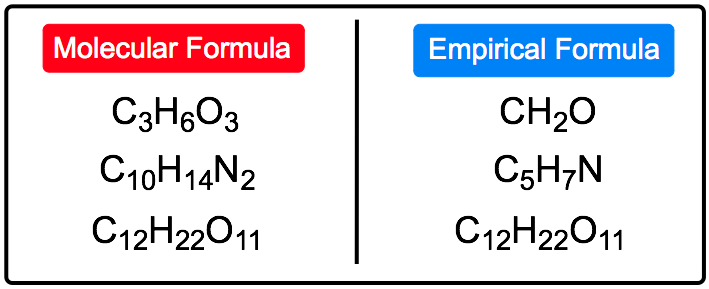Clutch Prep is now a part of Pearson
Ch.3 - Chemical ReactionsWorksheetSee all chapters

# Empirical Formula

See all sections
Sections
Empirical Formula
Molecular Formula
Combustion Analysis
Combustion Apparatus
Polyatomic Ions
Naming Ionic Compounds
Writing Ionic Compounds
Naming Ionic Hydrates
Naming Acids
Naming Molecular Compounds
Balancing Chemical Equations
Stoichiometry
Limiting Reagent
Percent Yield
Mass Percent
Functional Groups in Chemistry

The empirical formula gives the relative number of atoms.

###### Empirical Formula

Concept #1: Empirical FormulaExample #1: Determine the empirical formula of a compound that is 68.40% chromium and 31.60% oxygen.

Practice: A compound that contains only carbon, hydrogen, and oxygen is composed of 48.64% C and 43.2% O by mass. What is the empirical formula of this compound?

Practice: Elemental analysis of a sample of an ionic compound showed 2.82 g of Na, 4.35 g of Cl, and 7.83 g of O. What is the empirical formula of the compound?

Practice: A compound composed of carbon, hydrogen, and chlorine contains 4.19 x 1023 hydrogen atoms. If 9.00 g of the compound also contains 55.0% chlorine by mass, what is the empirical formula?